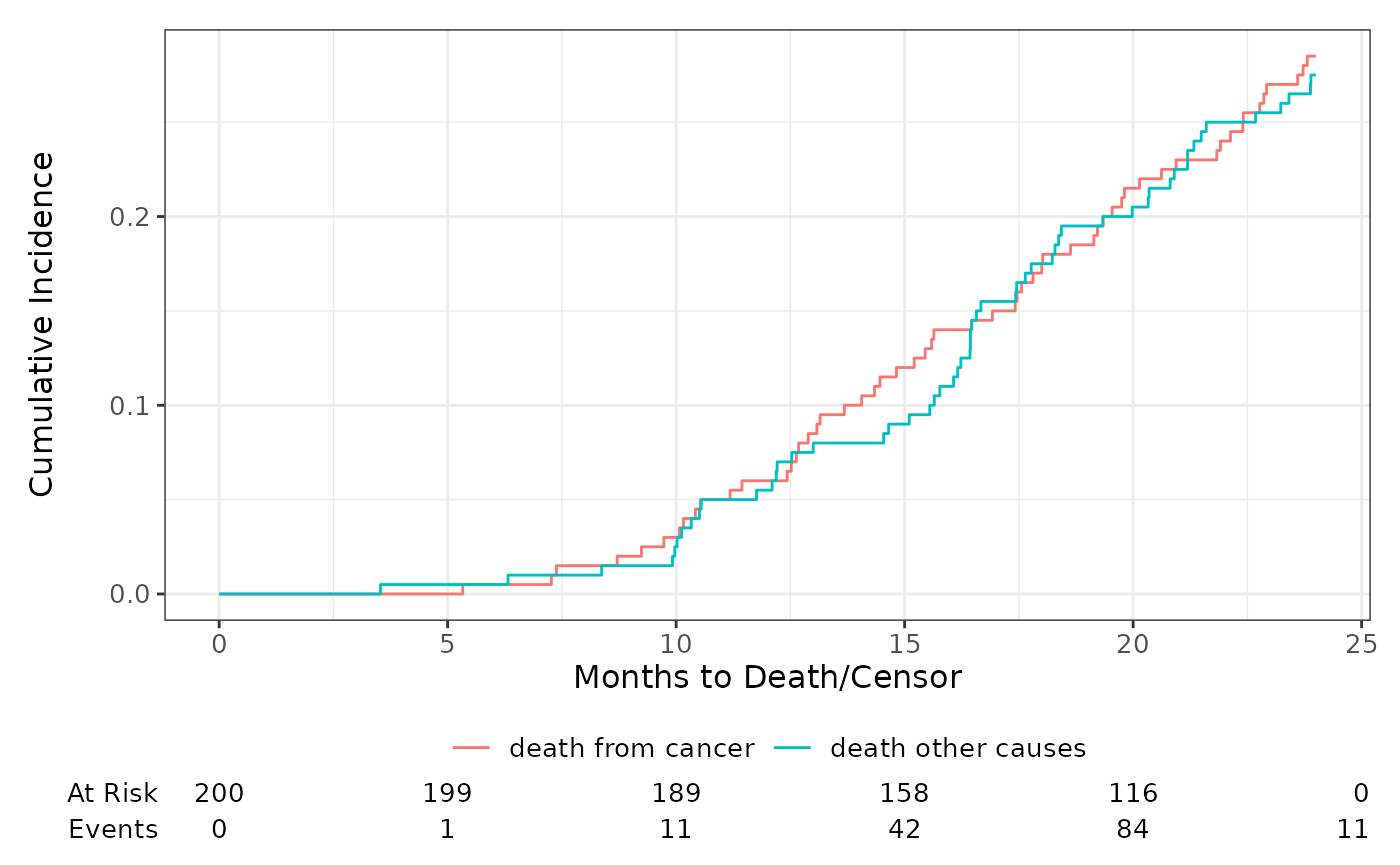The gallery exhibits both default plots as well as the many modifications one can make.

library(ggsurvfit)
library(patchwork)

## Modifications with ggplot2

Let’s begin with showing the default plot and common modifications that are made with ggplot2 functions.

• Expand axis to show percentages from 0% to 100%
• Limit plot to show up to 8 years of follow-up
• Add the percent sign to the y-axis label
• Reduce padding in the plot area around the curves
• Update color of the lines
• Using the ggplot2 minimal theme
gg_default <-
survfit2(Surv(time, status) ~ surg, data = df_colon) %>%
ggsurvfit() +
labs(title = "Default")

gg_styled <-
gg_default +
coord_cartesian(xlim = c(0, 8)) +
scale_y_continuous(
limits = c(0, 1),
labels = scales::percent,
expand = c(0.01, 0)
) +
scale_x_continuous(breaks = 0:9, expand = c(0.02, 0)) +
scale_color_manual(values = c('#54738E', '#82AC7C')) +
scale_fill_manual(values = c('#54738E', '#82AC7C')) +
theme_minimal() +
theme(legend.position = "bottom") +
guides(color = guide_legend(ncol = 1)) +
labs(
title = "Modified",
y = "Percentage Survival"
)

gg_default + gg_styled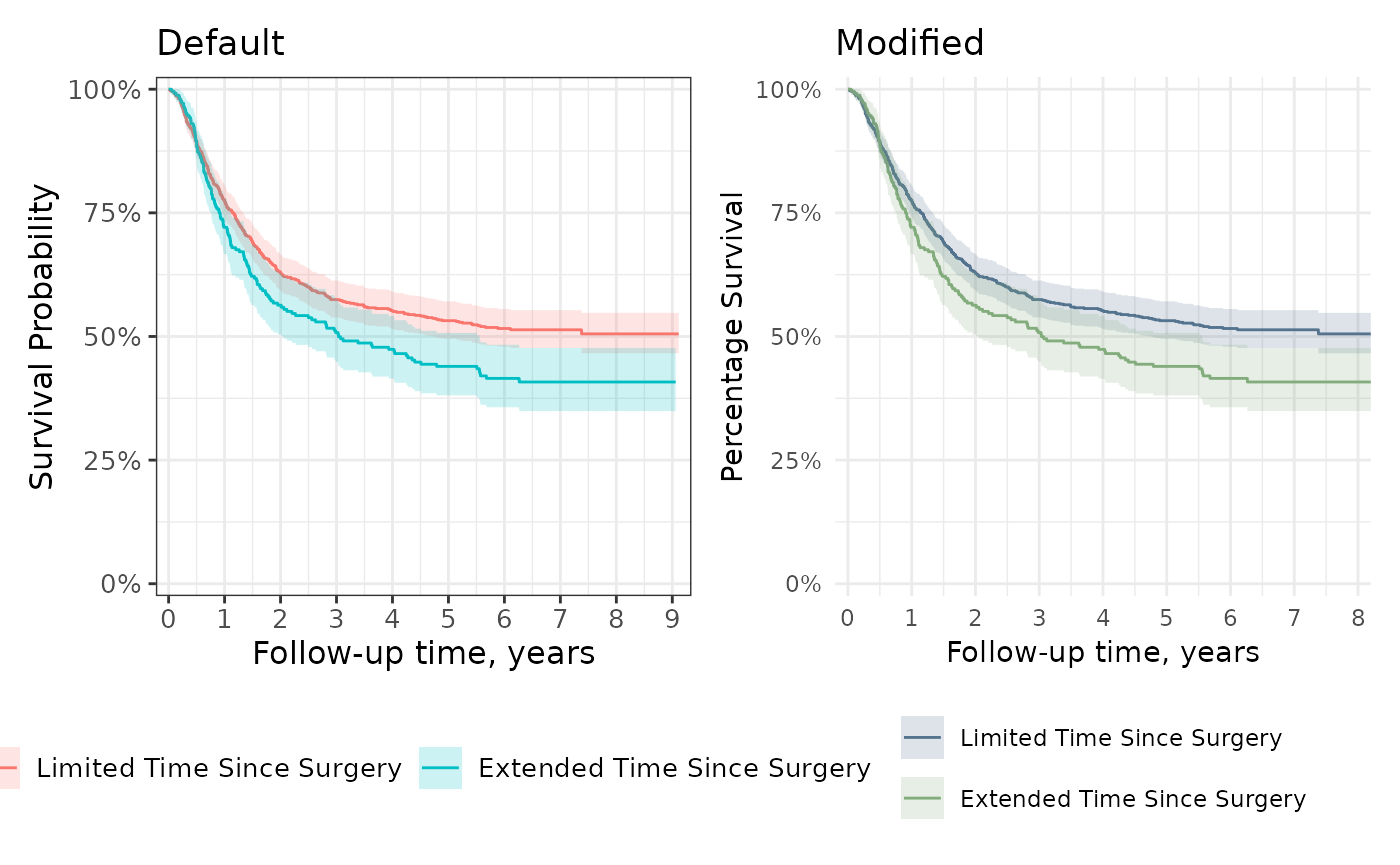In addition to using additional {ggplot2} functions, it is helpful to understand which underlying functions are used to create the figures. The survival lines are drawn with geom_step() and the confidence interval with geom_ribbon(). Users can pass additional arguments to these construction functions in ggsurvfti(...) and add_confidence_interval(...), respectively. In the example below, we use the color and fill aesthetic to change the colors of an unstratified estimate.

survfit2(Surv(time, status) ~ 1, data = df_colon) %>%
ggsurvfit(color = "#508050") +
scale_ggsurvfit()## Risk Tables

The default risk table styling is ready for publication.

survfit2(Surv(time, status) ~ surg, data = df_colon) %>%
ggsurvfit() +
add_risktable()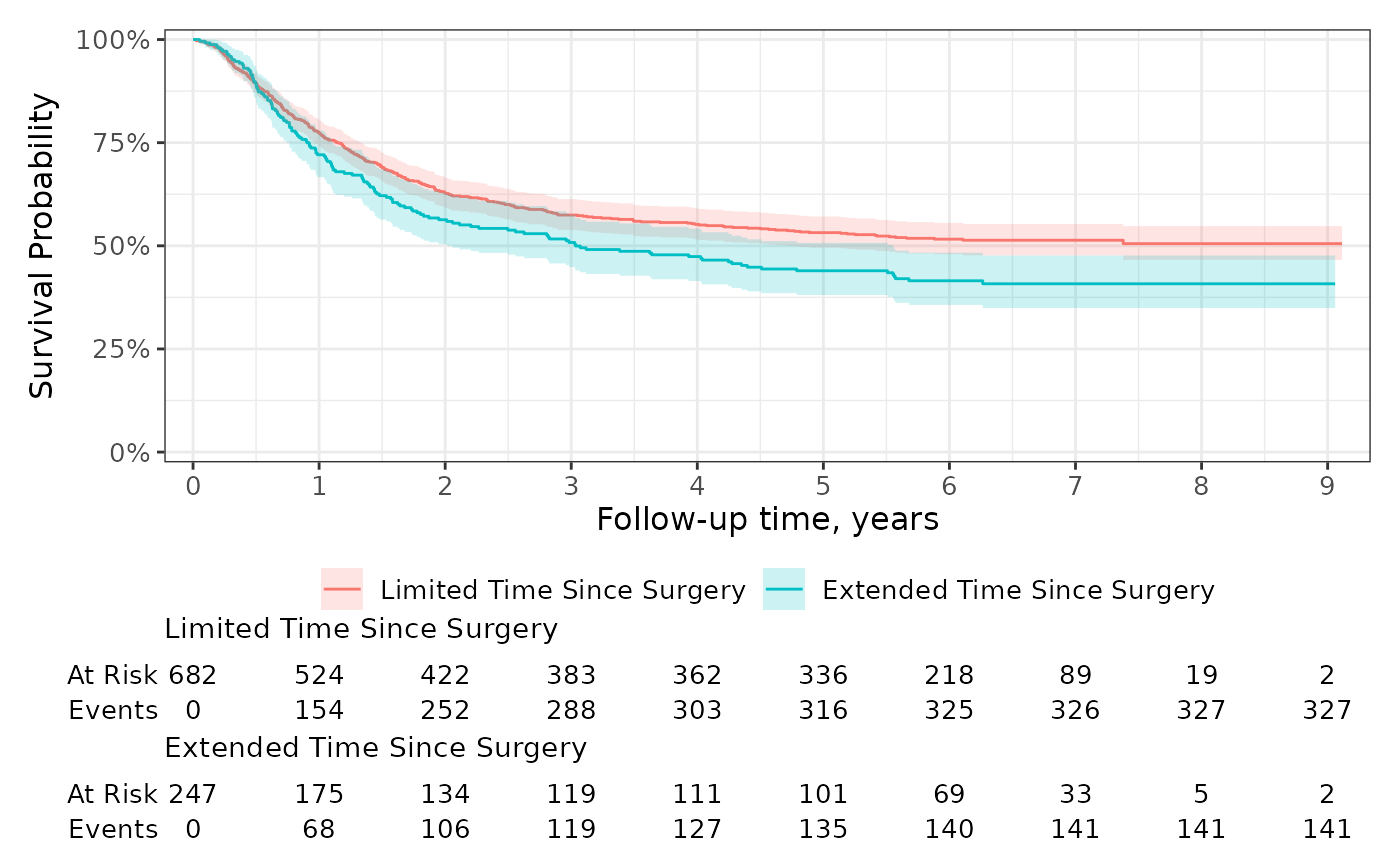You can also group the risk table by the statistics rather than the stratum. Let’s also add additional time points where the statistics are reported and extend the y axis.

ggrisktable <-
survfit2(Surv(time, status) ~ surg, data = df_colon) %>%
ggsurvfit() +
scale_x_continuous(breaks = 0:9) +
scale_y_continuous(limits = c(0, 1))
ggrisktableUse add_risktable_strata_symbol() to replace long stratum labels with a color symbol. The default symbol is a colored rectangle and you can change it to any UTF-8 symbol or text string. In the example below, we’ve updated the symbol to a circle.

ggrisktable +
add_risktable_strata_symbol(symbol = "\U25CF", size = 10)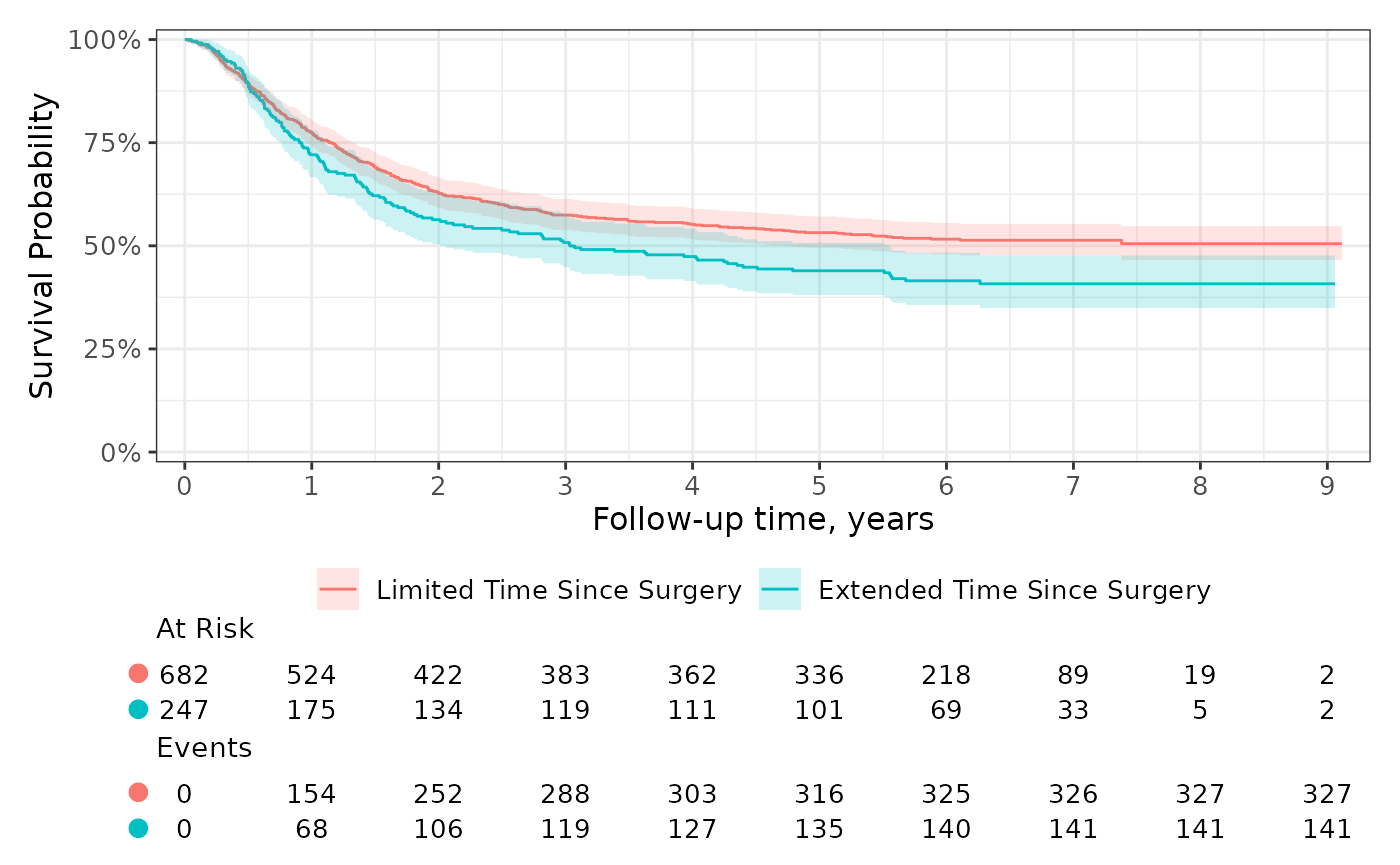You can further customize the risk table using themes and the add_risktable(...) arguments. For example, use the following code to increase the font size of both the risk table text and the y-axis label.

survfit2(Surv(time, status) ~ surg, data = df_colon) %>%
ggsurvfit(linewidth = 0.8) +
risktable_height = 0.33,
size = 4, # increase font size of risk table statistics
theme =   # increase font size of risk table title and y-axis label
list(
theme_risktable_default(axis.text.y.size = 11,
plot.title.size = 11),
theme(plot.title = element_text(face = "bold"))
)
)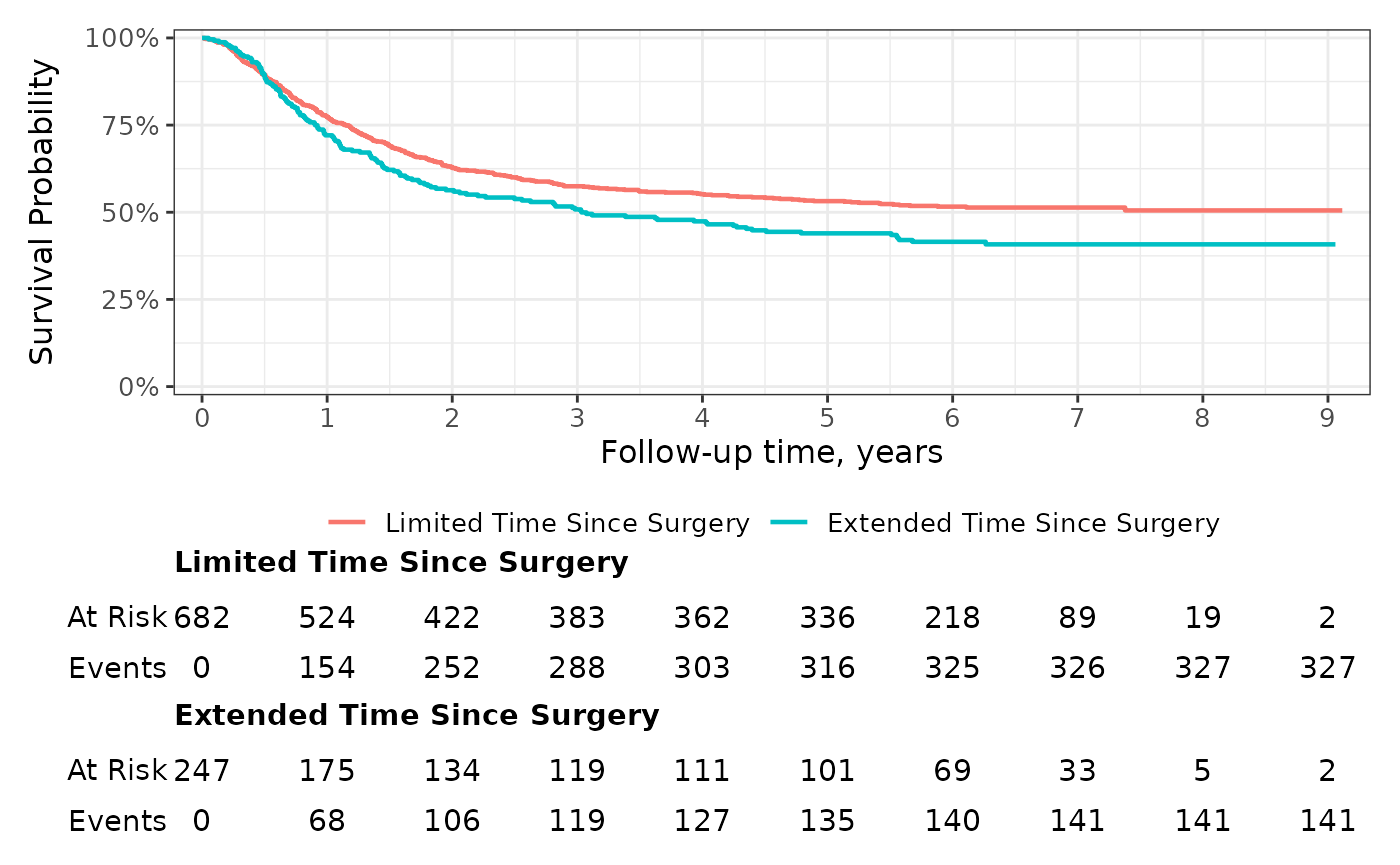You can also use glue-like syntax to place multiple statistics on the same row of the risk table.

survfit2(Surv(time, status) ~ sex, data = df_lung) %>%
ggsurvfit() +
add_risktable(risktable_stats = "{n.risk} ({cum.event})")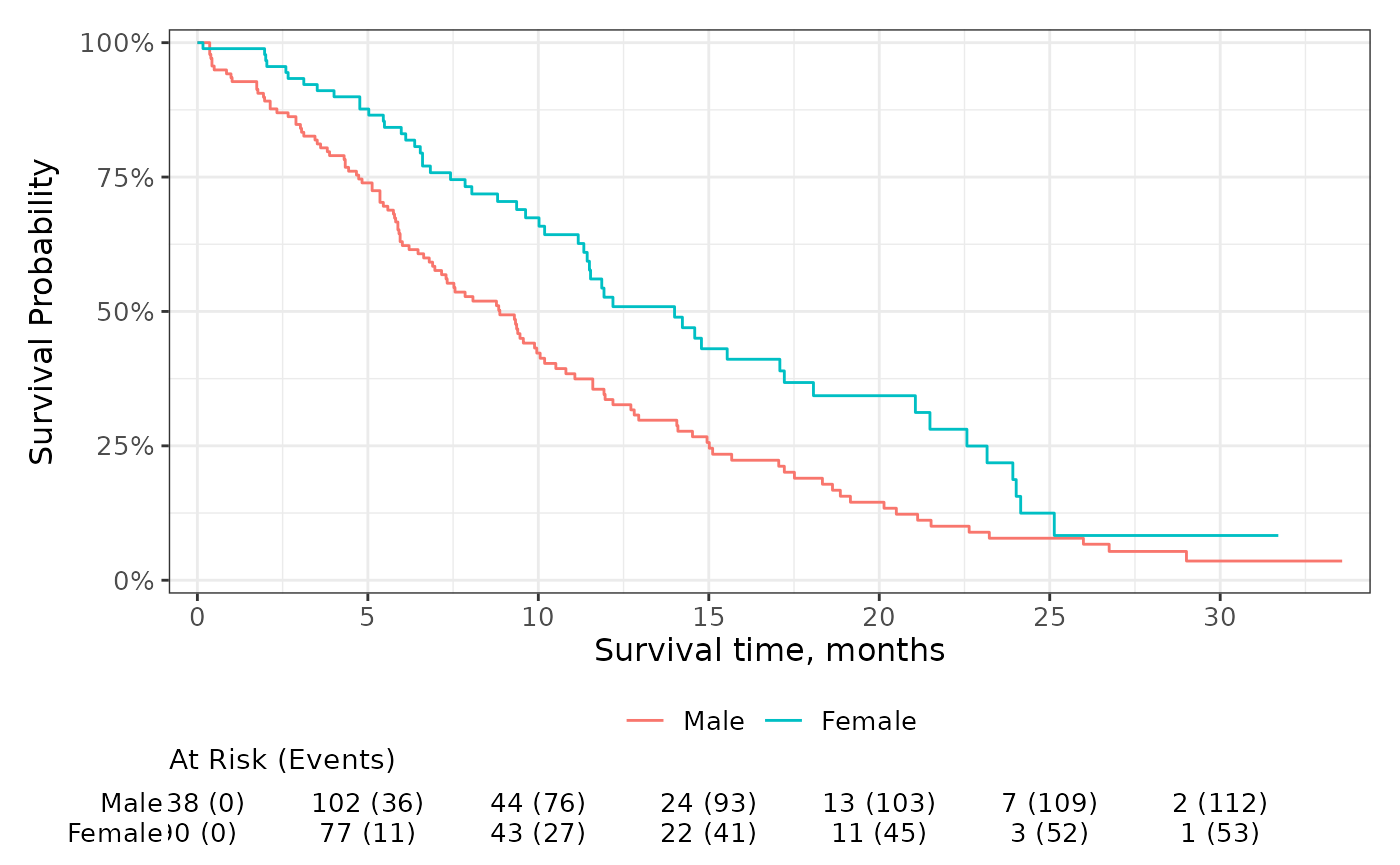## Quantiles and Censor Markings

Add guidelines for survival quantiles and markings for censored patients using add_quantile() and add_censor_mark(). The add_quantile() function allows users to place guidelines by specifying either the y-intercept or x-intercept where the lines shall originate.

survfit2(Surv(time, status) ~ surg, data = df_colon) %>%
ggsurvfit(linewidth = 0.8) +
add_censor_mark(size = 2, alpha = 0.2) +
add_quantile(y_value = 0.5, linetype = "dotted", color = "grey30", linewidth = 0.8) +
add_quantile(x_value = 5,  color = "grey30", linewidth = 0.8) +
scale_ggsurvfit()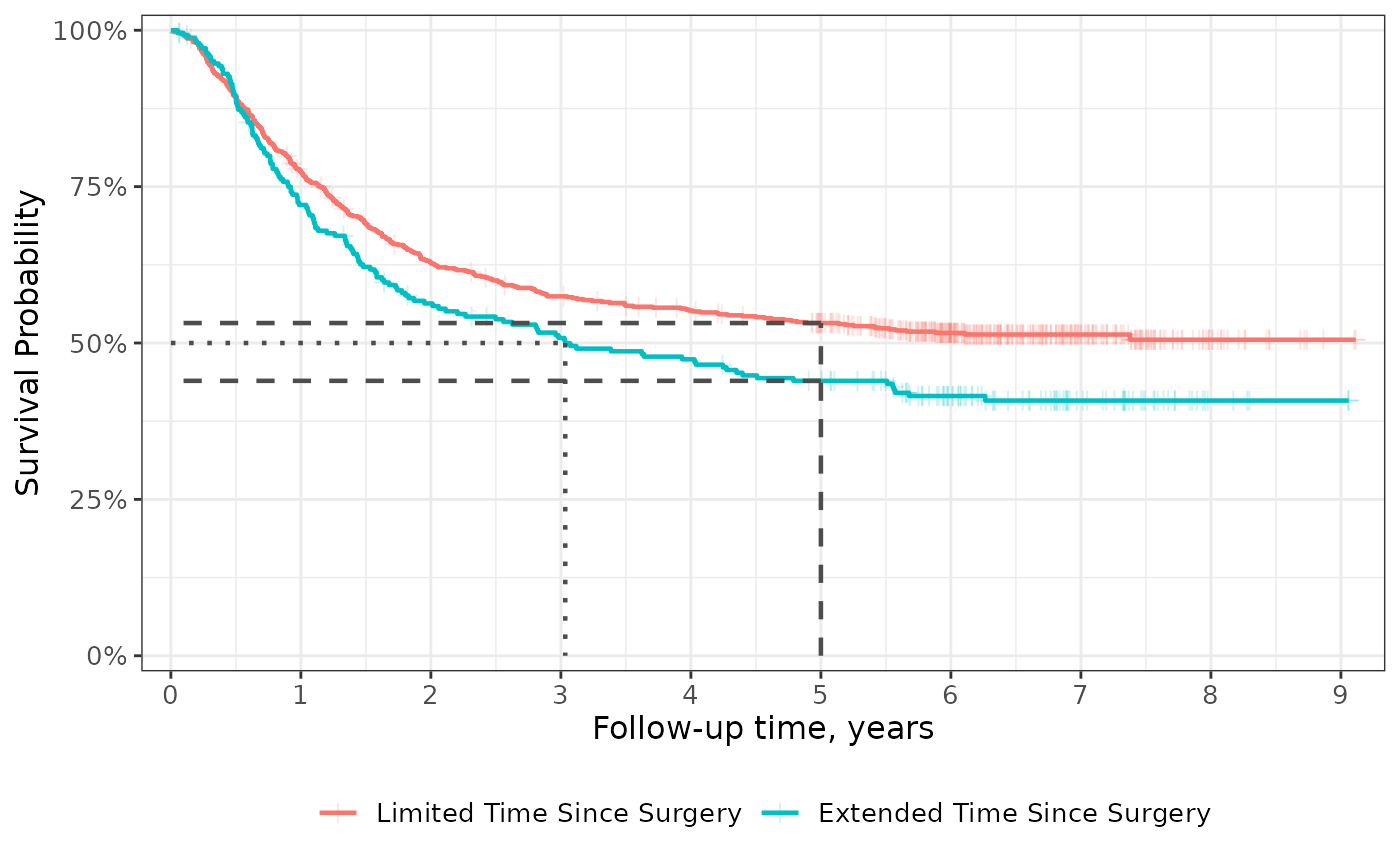## Side-by-Side

One of my favorite features of the {ggsurvfit} package is that any {ggplot2} function may be used to modify the plot and the risk tables will still align with the primary plot. This is accomplished by delaying the construction of the risk tables until the plot is printed.

Because the the delayed build of the risk tables, we must take one additional step when placing figures side-by-side that contain risk tables: we must use the ggsurvfit_build() function. This function will construct the risk tables and combine them with the primary plot. Once the plots are built, the can be placed side-by-side with {patchwork} or {cowplot}.

p <-
survfit2(Surv(time, status) ~ 1, df_colon) %>%
ggsurvfit() +
scale_y_continuous(limits = c(0, 1)) +
scale_x_continuous(n.breaks = 7)

# build plot (which constructs the risktable)
built_p <- ggsurvfit_build(p)

#### patchwork

Combine with patchwork::wrap_plots()

wrap_plots(built_p, built_p, ncol = 2)

To use patchwork plot arithmetic, each plot must be wrapped in patchwork::wrap_elements()

wrap_elements(built_p) | wrap_elements(built_p)

#### cowplot

Combine with cowplot::plot_grid()

cowplot::plot_grid(built_p, built_p, ncol = 2)## P-values

Compare curves among the stratum using the add_p() function. P-values can be placed either in the figure caption (the default) or in the plot area as an annotation.

p <- survfit2(Surv(time, status) ~ surg, data = df_colon) %>%
ggsurvfit()

# place p-value in caption
p1 <- p + add_pvalue(caption = "Log-rank {p.value}")

# place p-value as a plot annotation
p2 <- p + add_pvalue(location  = "annotation", x = 8.5)

p1 + p2 +
patchwork::plot_layout(guides = "collect") &
theme(legend.position = "bottom")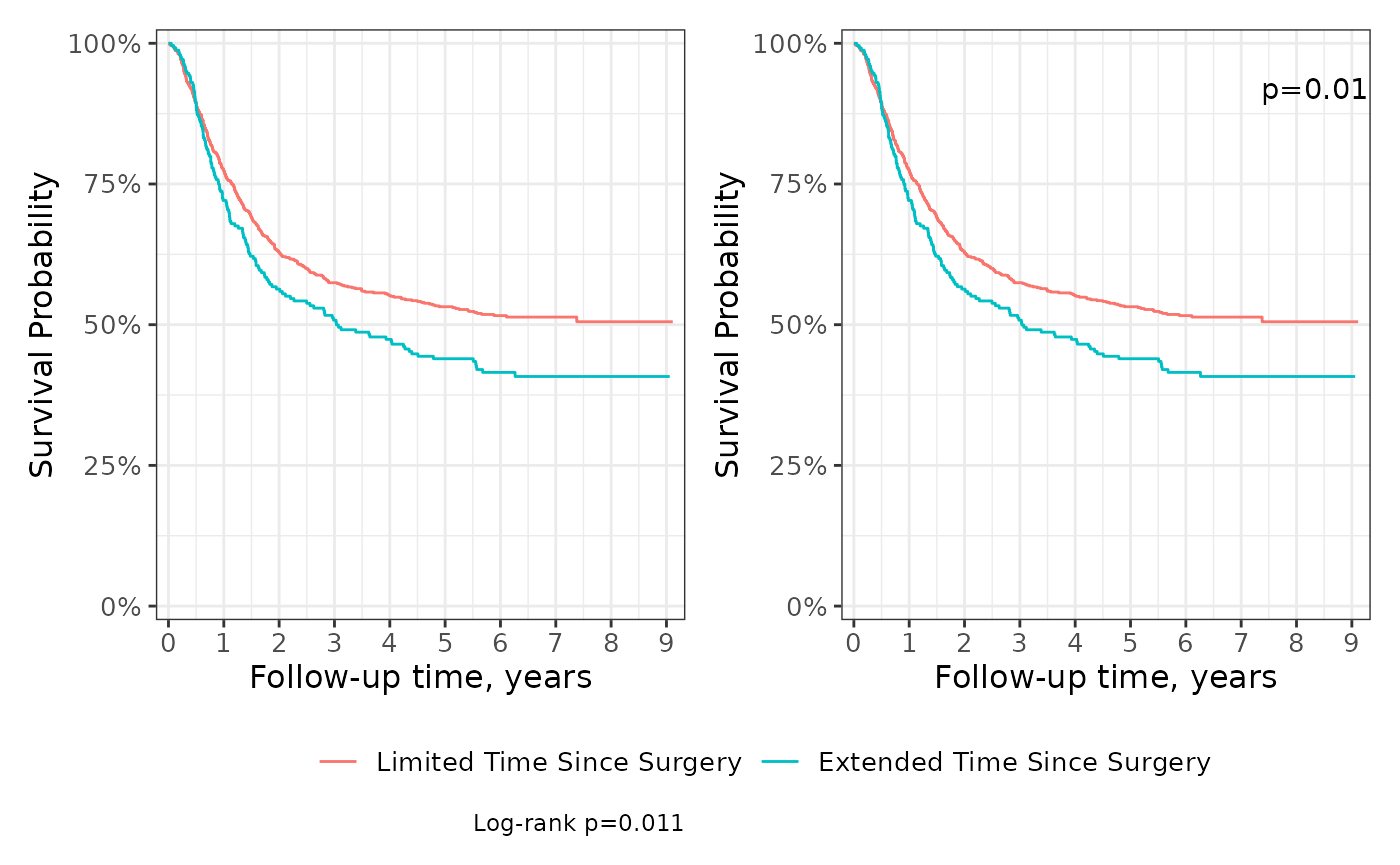## Scales

Unlike most {ggplot2} functions, scales are not additive. This means that if a scale attribute is modified in one call to scale_x_continuous(), a second call to scale_x_continuous() will write over all changes made in the first. For this reason, the ggsurvfit() and ggcuminc() functions do not modify the default {ggplot2} scales; rather, all changes to the scales are left to the user. But, we do export a helpful ggsurvfit-specific scales function to help.

The scale_ggsurvfit() function will apply default scales often seen in survival figures: reduced plot padding, y-axis labels appear as percentages, survival curves are shown from 0% to 100% on the y-axis. In the example below, we utilize scale_ggsurvfit() and make other scale changes to the scales, such as, specifying the breaks on the x-axis.

survfit2(Surv(time, status) ~ surg, data = df_colon) %>%
ggsurvfit(linewidth = 1) +
scale_ggsurvfit(x_scales = list(breaks = 0:9))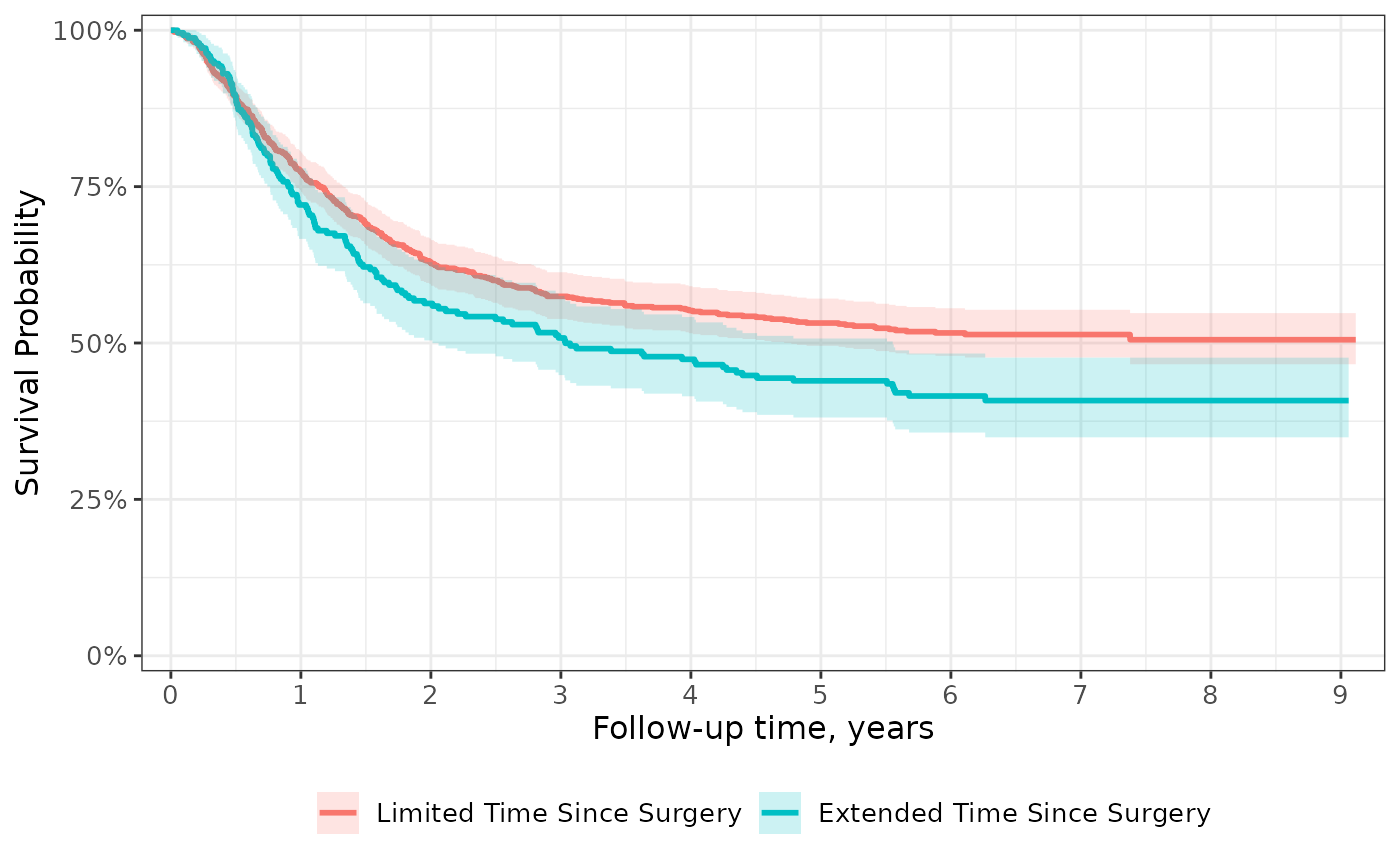## Transformations

Show the probability of an event rather than the probability of being free from the event with transformations. Custom transformations are also available.

p <-
survfit2(Surv(time, status) ~ surg, data = df_colon) %>%
ggsurvfit(type = "risk", linewidth = 0.8) +
p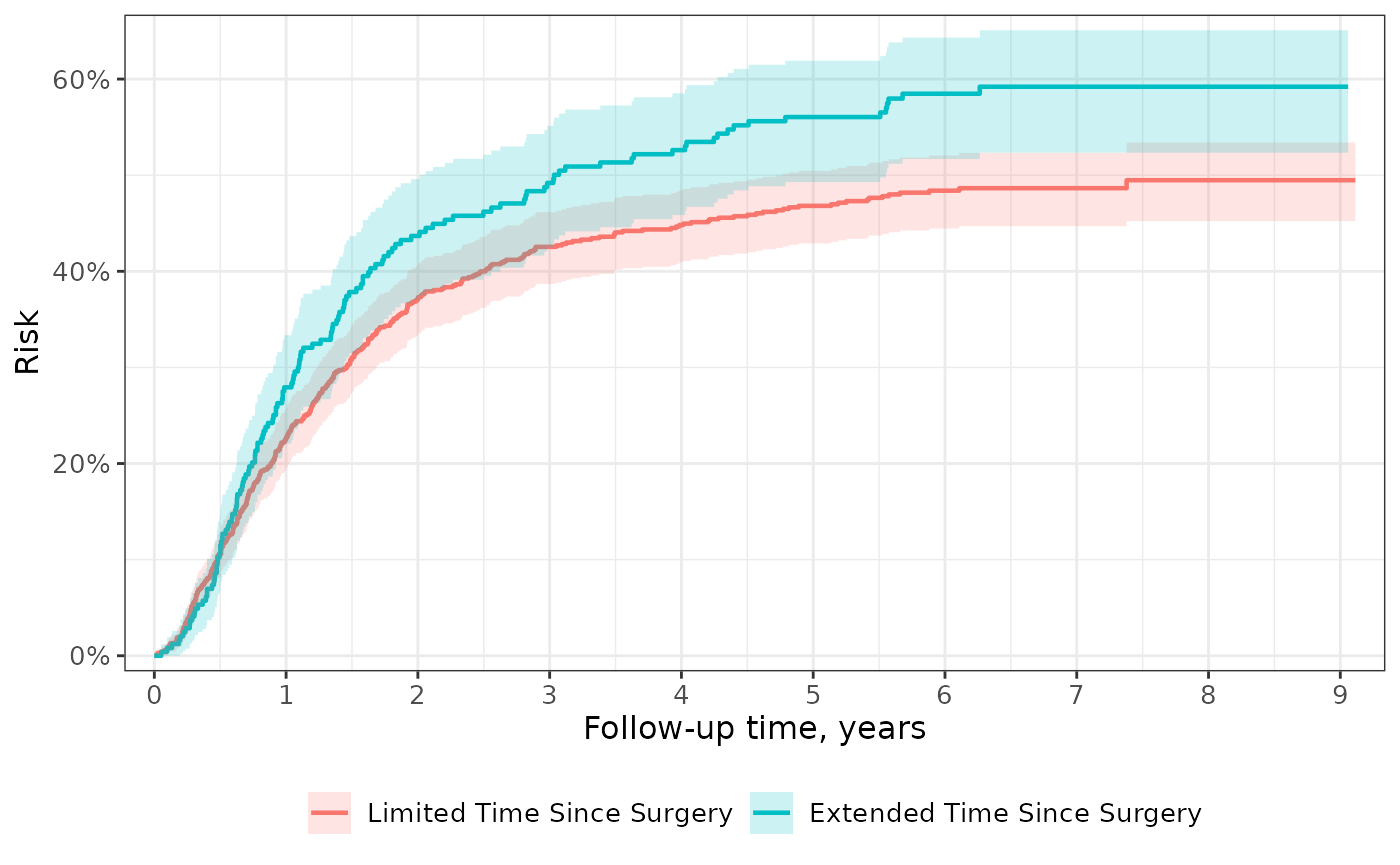## Saving Plots

The {ggsurvfit} package plays well with ggplot2::ggsave(), which allows you to specify the output file format, the DPI, the height and width of a the image, and more.

path_to_image <- file.path(tempdir(), "image.png")
path_to_image
#>  "/tmp/RtmpNKlHFa/image.png"

ggsave(file = path_to_image, plot = p)
#> Saving 7.29 x 4.51 in image

## Extensions

Because {ggsurvfit} functions are written as proper {ggplot2} geoms, you can both weave any {ggplot2} functions and ggplot2 extensions, such as {gghighlight} and {ggeasy}.

survfit2(Surv(time, status) ~ rx, data = df_colon) %>%
ggsurvfit(linewidth = 1) +
gghighlight::gghighlight(
strata == "Levamisole+5-FU",
calculate_per_facet = TRUE
) +
ggeasy::easy_remove_legend() +
ggeasy::easy_y_axis_labels_size(size = 15) +
ggeasy::easy_x_axis_labels_size(size = 15) +
ggeasy::easy_y_axis_title_size(size = 15) +
ggeasy::easy_x_axis_title_size(size = 15)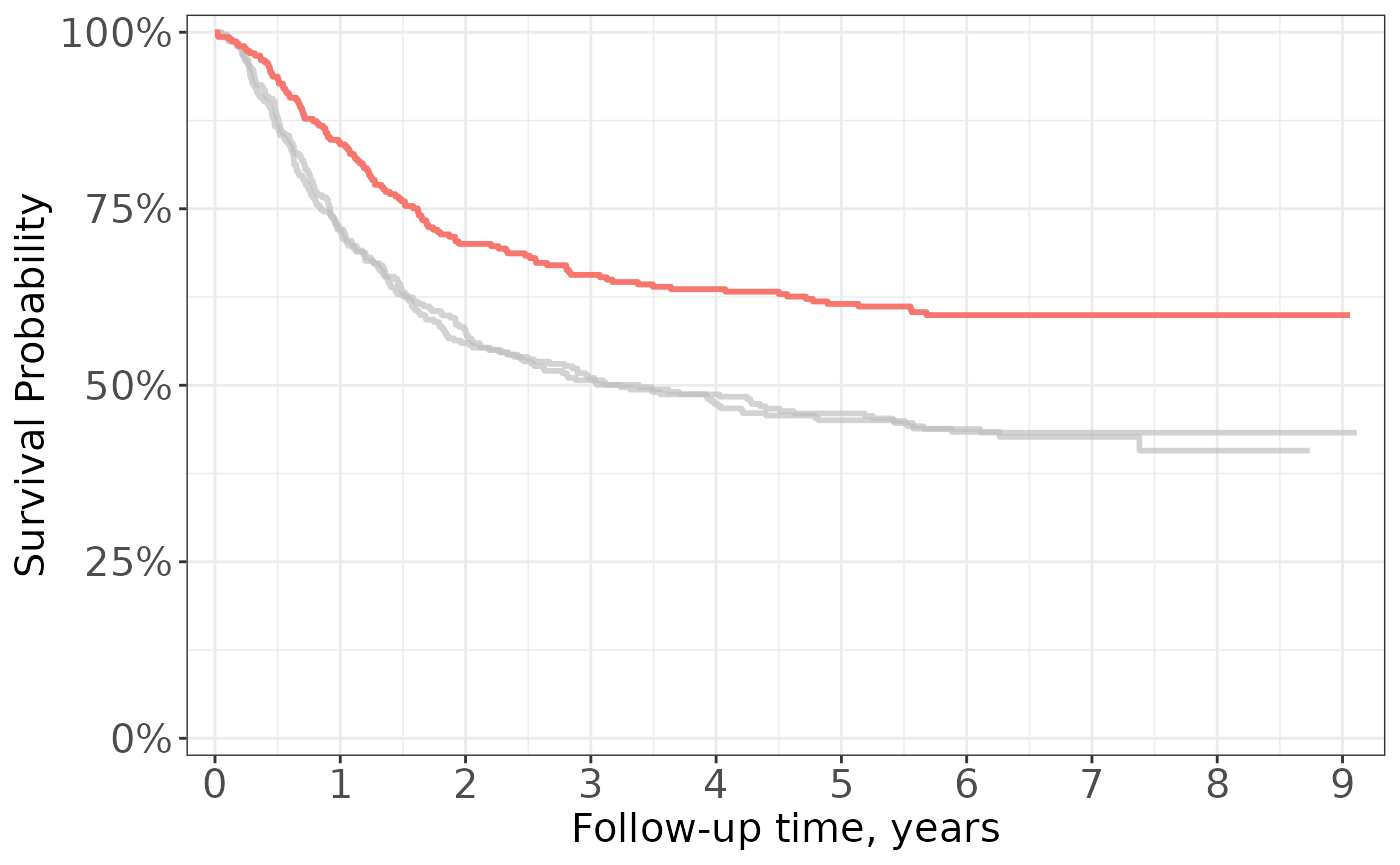## Faceting

Curves created with {ggsurvfit} can also later be faceted using {ggplot2}. Note, however, that faceted curves cannot include a risk table.

The ggsurvfit() function calls tidy_survfit() to create the data frame that is used to create the figure. In the data frame, there is a column named "strata", which we will facet over.

survfit2(Surv(time, status) ~ surg, data = df_colon) %>%
ggsurvfit() +
facet_wrap(~strata, nrow = 1) +
theme(legend.position = "none") +
scale_x_continuous(n.breaks = 6) +
labs(title = "PFS by Duration between Surgery and Treatment")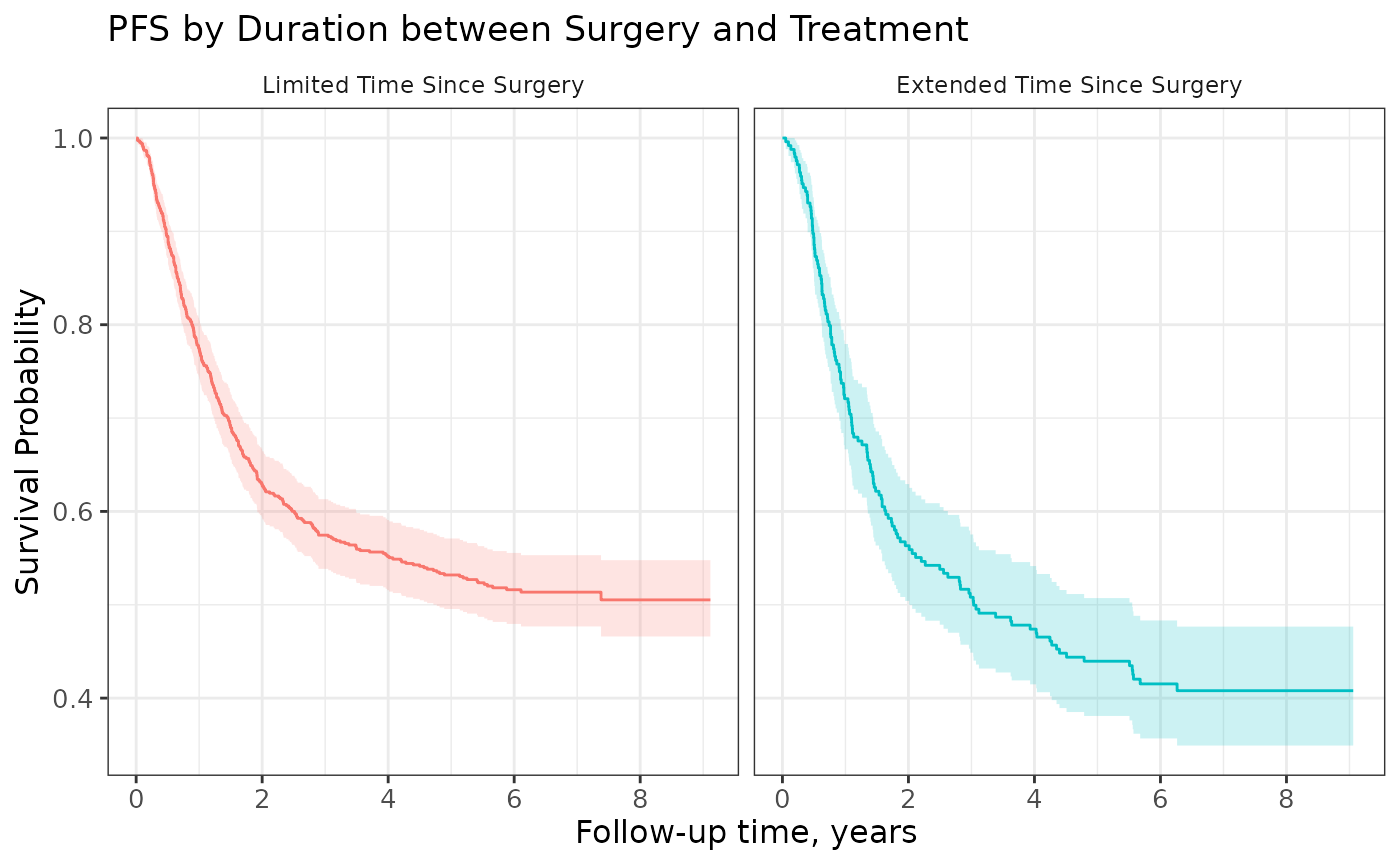## Grey-scale Figures

You may need a black and white figure and that is achieved using grey-scale ggplot2 functions.

survfit2(Surv(time, status) ~ surg, data = df_colon) %>%
ggsurvfit() +
scale_color_grey() +
scale_fill_grey() +
labs(title = "Grey Scale")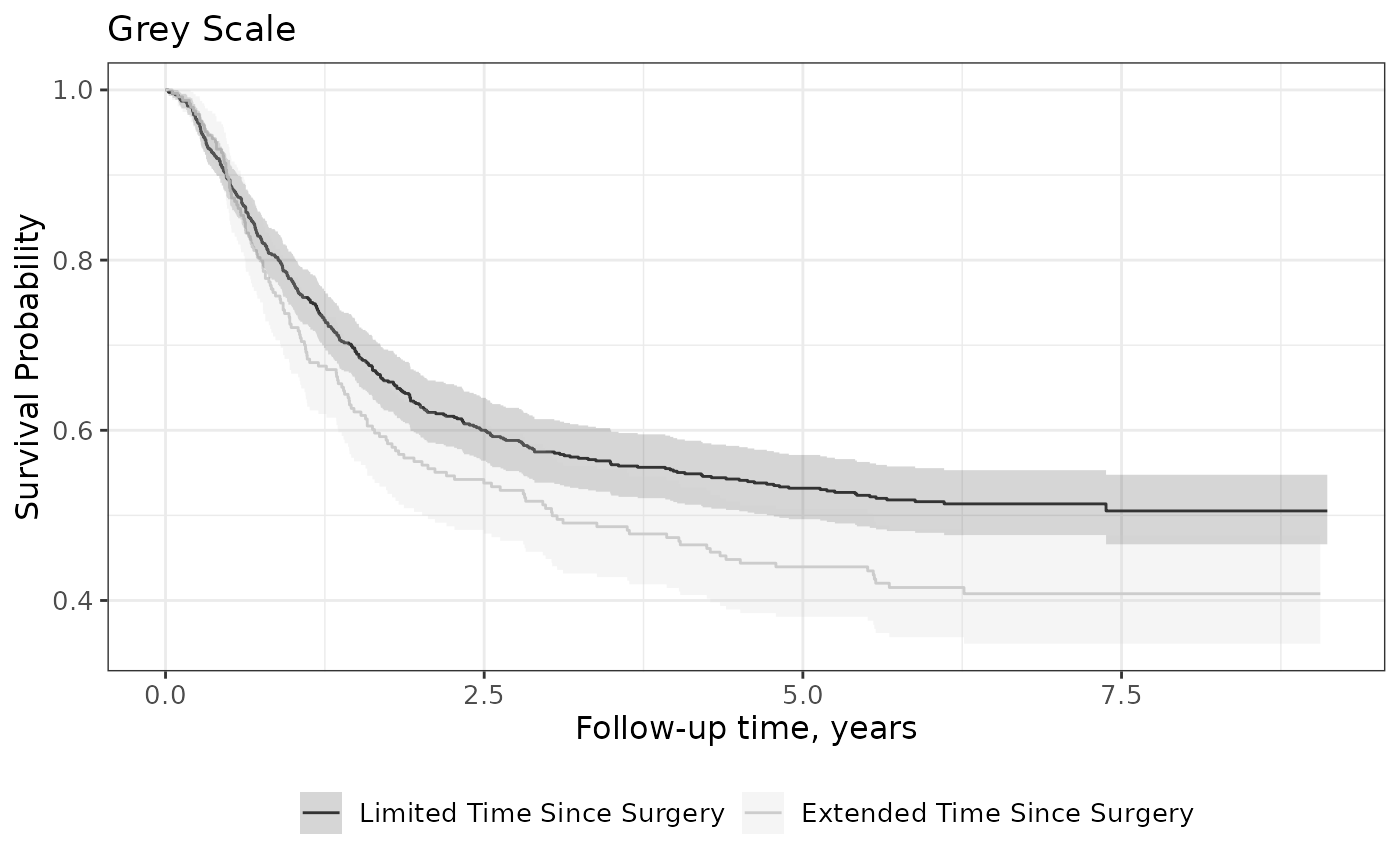## KMunicate

To get figures that align with the guidelines outlined in “Proposals on Kaplan–Meier plots in medical research and a survey of stakeholder views: KMunicate.”, use the theme_ggsurvfit_KMunicate() theme along with these function options.

survfit2(Surv(time, status) ~ surg, data = df_colon) %>%
ggsurvfit(linetype_aes = TRUE) +
risktable_stats = c("n.risk", "cum.censor", "cum.event")
) +
theme_ggsurvfit_KMunicate() +
scale_y_continuous(limits = c(0, 1)) +
scale_x_continuous(expand = c(0.02, 0)) +
theme(legend.position = c(0.85, 0.85))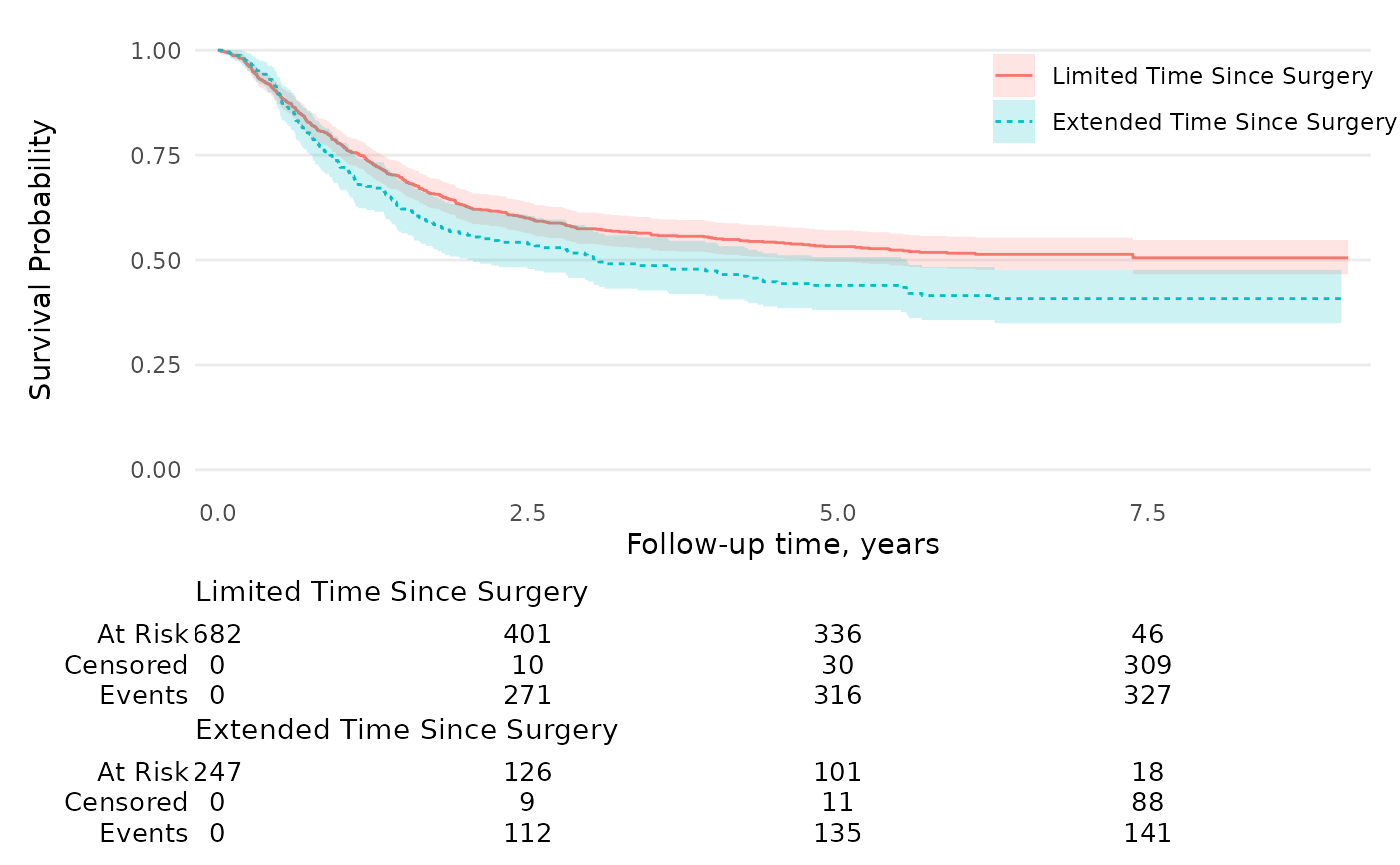The ggsurvfit supports printing adjusted Cox Proportion Hazards Regression models. Any stratifying levels must be wrapped in a strata() call in the RHS of the formula.
library(survival)
scale_y_continuous(limits = c(0, 1))